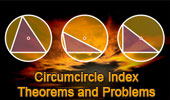Plane Geometry: Circumcircle, Circumradius of a triangle, Theorems and Problems 1

 Circumcircle of a triangle - Table of Content 1Distances between Triangle Centers Index. Nine-Point Center, Nine-Point Circle, Euler Line (English version). Circumcenter, Centroid, Orthocenter, Circumcircle Interactive illustration. Poly for iPad. Polygonal Art, Delaunay Triangulation. Lemoine Line Theorem, Interactive illustration. HTML5 Animation for iPad and Nexus GeoGebra, Dynamic Geometry: Lemoine Line of a Triangle. HTML5 Animation for Tablets (iPad, Nexus..) GeoGebra, Dynamic Geometry: Simson Line of a Triangle. HTML5 Animation for Tablets (iPad, Nexus..) Dynamic Geometry 1448. Simson Line. Step-by-step illustration using GeoGebra. Dynamic Geometry 1448. Simson Line. Step-by-step illustration using GeoGebra. Dynamic Geometry 1448. Simson Line. Step-by-step illustration using GeoGebra. Dynamic Geometry Problem 1446. Lemoine Line, triangle, circumcircle, tangent, collinear points. Step-by-step animation using GeoGebra. Geometry Problem 1005. Triangle, Circle, Circumcenter, Interior and Exterior Angle Bisector, 90 Degree, Perpendicular. Geometry Problem 1003. Intersecting Circle, Circumcircle, Chord, Secant, Triangle, 90 Degree, Angle, Perpendicular Lines. Geometry Problem 1002. Triangle, Circumcircle, Cevian, Parallel Lines, Cyclic Quadrilateral, Concyclic Points. Geometry Problem 1001. Triangle, Circumcircle, Perpendicular, Perpendicular Bisector, Tangent, Collinear Points. Geometry Problem 987. Triangle, Circumcircle, Incenter, Chord, Parallel, Circle, Tangent. GeoGebra, HTML5 Animation for Tablets (iPad, Nexus). Geometry Problem 950. Intersecting Circles, Secant, Cyclic Quadrilateral, Concyclic Points. Go to page: Previous | 1 | 2 | 3 | 4 | 5 | 6 | 7 | 8 | Next

 Home | Search| Geometry | Triangles | Centers | Post a comment | Email | by Antonio Gutierrez